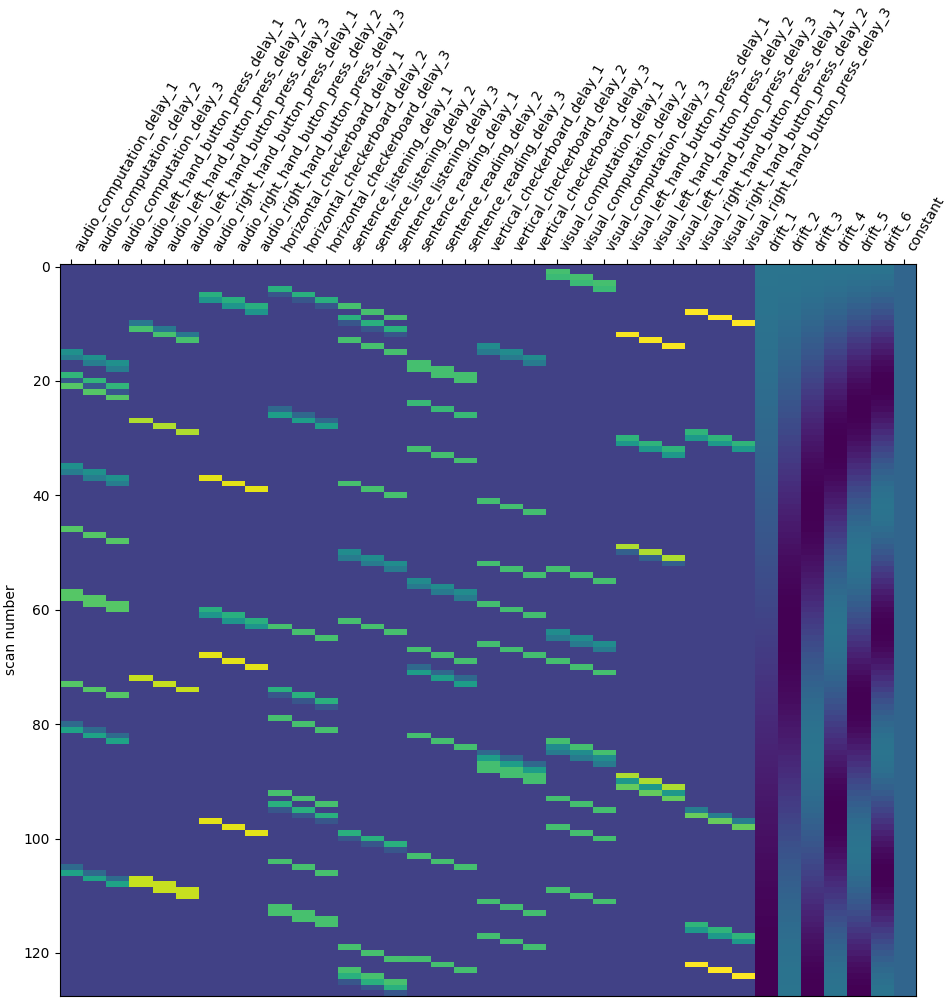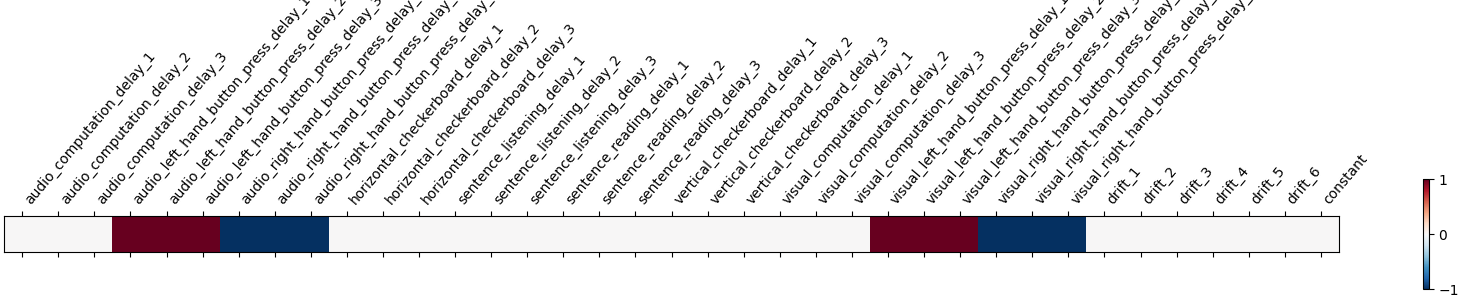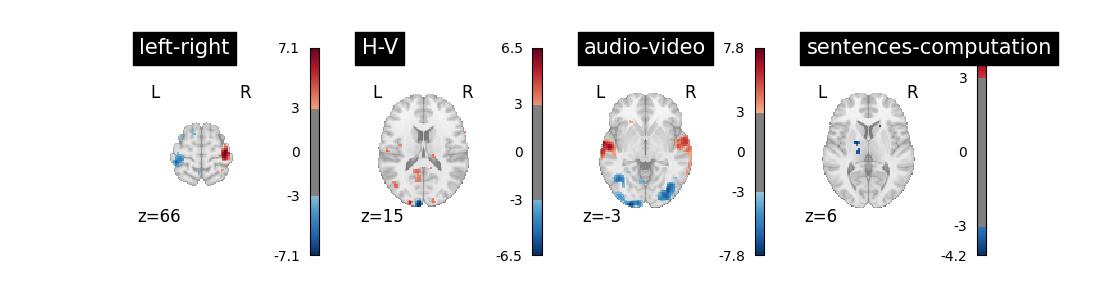# Analysis of an fMRI dataset with a Finite Impule Response (FIR) model#

FIR models are used to estimate the hemodyamic response non-parametrically. The example below shows that they’re good to do statistical inference even on fast event-related fMRI datasets.

Here, we demonstrate the use of a FIR model with 3 lags, computing 4 contrasts from a single subject dataset from the “Neurospin Localizer”. It is a fast event related design: During 5 minutes, 80 events of the following types are presented : [‘audio_computation’, ‘audio_left_hand_button_press’, ‘audio_right_hand_button_press’, ‘horizontal_checkerboard’, ‘sentence_listening’, ‘sentence_reading’, ‘vertical_checkerboard’, ‘visual_computation’, ‘visual_left_hand_button_press’, ‘visual_right_hand_button_press’]

At first, we grab the localizer data.

```import pandas as pd
from nilearn.datasets import func

data = func.fetch_localizer_first_level()
fmri_img = data.epi_img
t_r = 2.4
events_file = data["events"]
```

Next solution is to try Finite Impulse Response (FIR) models: we just say that the hrf is an arbitrary function that lags behind the stimulus onset. In the present case, given that the numbers of conditions is high, we should use a simple FIR model.

Concretely, we set hrf_model to ‘fir’ and fir_delays to [1, 2, 3] (scans) corresponding to a 3-step functions on the [1 * t_r, 4 * t_r] seconds interval.

```from nilearn.glm.first_level import FirstLevelModel
from nilearn.plotting import plot_contrast_matrix, plot_design_matrix

first_level_model = FirstLevelModel(t_r, hrf_model="fir", fir_delays=[1, 2, 3])
first_level_model = first_level_model.fit(fmri_img, events=events)
design_matrix = first_level_model.design_matrices_
plot_design_matrix(design_matrix)
``````<AxesSubplot:label='conditions', ylabel='scan number'>
```

We have to adapt contrast specification. We characterize the BOLD response by the sum across the three time lags. It’s a bit hairy, sorry, but this is the price to pay for flexibility…

```import numpy as np

contrast_matrix = np.eye(design_matrix.shape)
contrasts = {
column: contrast_matrix[i]
for i, column in enumerate(design_matrix.columns)
}
conditions = events.trial_type.unique()
for condition in conditions:
contrasts[condition] = np.sum(
[
contrasts[name]
for name in design_matrix.columns
if name[: len(condition)] == condition
],
0,
)

contrasts["audio"] = np.sum(
[
contrasts[name]
for name in [
"audio_right_hand_button_press",
"audio_left_hand_button_press",
"audio_computation",
"sentence_listening",
]
],
0,
)
contrasts["video"] = np.sum(
[
contrasts[name]
for name in [
"visual_right_hand_button_press",
"visual_left_hand_button_press",
"visual_computation",
]
],
0,
)

contrasts["computation"] = (
contrasts["audio_computation"] + contrasts["visual_computation"]
)
contrasts["sentences"] = (
)

contrasts = {
"left-right": (
contrasts["visual_left_hand_button_press"]
+ contrasts["audio_left_hand_button_press"]
- contrasts["visual_right_hand_button_press"]
- contrasts["audio_right_hand_button_press"]
),
"H-V": (
contrasts["horizontal_checkerboard"]
- contrasts["vertical_checkerboard"]
),
"audio-video": contrasts["audio"] - contrasts["video"],
"sentences-computation": (
contrasts["sentences"] - contrasts["computation"]
),
}
```

Take a look at the contrasts.

```plot_contrast_matrix(contrasts["left-right"], design_matrix)
``````<AxesSubplot:label='conditions'>
```

Take a breath.

We can now proceed by estimating the contrasts and displaying them.

```import matplotlib.pyplot as plt
from nilearn.plotting import plot_stat_map

fig = plt.figure(figsize=(11, 3))
for index, (contrast_id, contrast_val) in enumerate(contrasts.items()):
ax = plt.subplot(1, len(contrasts), 1 + index)
z_map = first_level_model.compute_contrast(
contrast_val, output_type="z_score"
)
plot_stat_map(
z_map,
display_mode="z",
threshold=3.0,
title=contrast_id,
axes=ax,
cut_coords=1,
)
plt.show()
```The result is acceptable. Note that we’re asking a lot of questions to a small dataset, yet with a relatively large number of experimental conditions.

Total running time of the script: ( 0 minutes 18.041 seconds)

Estimated memory usage: 259 MB

Gallery generated by Sphinx-Gallery